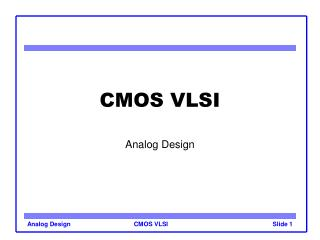DownloadDownload PresentationCMOS VLSI

# CMOS VLSI

Download Presentation## CMOS VLSI

- - - - - - - - - - - - - - - - - - - - - - - - - - - E N D - - - - - - - - - - - - - - - - - - - - - - - - - - -
##### Presentation Transcript

1. CMOS VLSI Analog Design Analog Design

2. Outline • Overview • Small signal model, biasing • Amplifiers • Common source, CMOS inverter • Current mirrors, Differential pairs • Operational amplifier • Data converters • DAC, ADC • RF • LNA, mixer Analog Design

3. CMOS for Analog • MOS device can be used for amplification as well as switching • Typical: operate devices in saturation, gate voltage sets current • Benefits • Cheap processes (compared to BJT) • Integrated packages • Challenges • Low gain • Coupling issues • Tolerances Analog Design

4. MOS Small Signal Model Analog Design

5. MOS Small Signal Model • From first order saturation equations: • Rewrite in terms of sensitivities: • So Analog Design

6. Channel Length Modulation • In reality output current does change with Vds • Output resistance Analog Design

7. Bias Point • Standard circuits for biasing • Compute parameters from I-V curves Analog Design

8. Outline • Overview • Small signal model, biasing • Amplifiers • Common source, CMOS inverter • Current mirrors, Differential pairs • Operational amplifier • Data converters • DAC, ADC • RF • LNA, mixer Analog Design

9. Common Source Amplifier • Operate MOS in saturation • Increase in Vgs leads to drop in vout • Gain A = vout/vin Analog Design

10. CMOS Inverter as an Amplifier • Can use pMOS tied to Vdd for resistive load in common source amplifier • Do better by having an “active load”: increase load resistance when Vin goes up Analog Design

11. AC Coupled CMOS Inverter • How to get maximum amplification? • Bias at Vinv using feedback resistor • Use capacitor to AC couple the input Analog Design

12. AC Coupled CMOS Inverter Analog Design

13. Current Mirrors • Replicate current at input at output • Ideally, Iout = Iin in saturation, so infinite output impedance • Channel length modulation: use large L Analog Design

14. Cascoded Current Mirror • Key to understanding: N1 and N2 have almost same drain and gate voltage • Means high output impedance Raise output impedance using a cascoded current mirror Analog Design

15. Current Mirror • Can use multiple output transistors to create multiple copies of input current • Better than using a single wider transistor, since identical transistors match better Analog Design

16. Differential Pair • Steers current to two outputs based on difference between two voltages • Common mode noise rejection Analog Design

17. Differential Amplifier • Use resistive loads on differential pair to build differential amplifier Analog Design

18. CMOS Opamp • Differential amplifier with common source amplifier • Diff amp uses pMOS current mirror as a load to get high impedance in a small area • Common source amp is P3, loaded by nMOS current mirror N5 • Bias voltage and current set by N3 and R • A = vo / (v2 – v1) = gmn2 gmp3 (ron2 | rop2) (rop3 | ron5) Opamp: workhorse of analog design Analog Design

19. Outline • Overview • Small signal model, biasing • Amplifiers • Common source, CMOS inverter • Current mirrors, Differential pairs • Operational amplifier • Data converters • DAC, ADC • RF • LNA, mixer Analog Design

20. Data Converters • DACs pretty easy to design, ADCs harder • Speed, linearity, power, size, ease-of-design • Parameters • Resolution, FSR • Linearity: DNL, INL, Offset Analog Design

21. Noise and Distortion Measures • DAC: apply digital sine wave, measure desired signal energy to harmonics and noise • ADC: apply analog sine wave, do FFT on the stored samples • Measure total harmonic distortion (THD), and spurious free dynamic range (SFDR) Analog Design

22. DAC • Resistor String DACs • Use a reference voltage ladder consisting of 2N resistors from VDD to GND for an N-bit DAC • Presents large RC, needs high load resistance • Use: reference for opamp, buffer, comparator Analog Design

23. DAC • R-2R DACs • Conceptually, evaluating binary expression • Much fewer resistors than resistor string DACs Analog Design

24. DAC • Current DAC: fastest converters • Basic principle • Different architectures Analog Design

25. DAC • Full implementation: 4-bit current DAC Analog Design

26. ADC • Speed of conversion, number of bits (¹ ENOBs) • Easy ADC: Successive Approximation Analog Design

28. ADC • Crucial components: comparator, encoder Analog Design

29. ADC • Pipeline ADC • Amounts to a distributed successive approx ADC • Trades flash speed and low latency for longer latency and slightly lower speed • Much less power Analog Design

30. ADC • Sigma-delta converter • Suitable for processes where digital is cheap • CD players: audio frequencies, 20 bit precision • RF (10MHz): 8-10 bit precision Analog Design

31. Outline • Overview • Small signal model, biasing • Amplifiers • Common source, CMOS inverter • Current mirrors, Differential pairs • Operational amplifier • Data converters • DAC, ADC • RF • LNA, mixers Analog Design

32. RF • Low in device count, very high in effort • Sizing, component selection very involved Analog Design

33. Mixers • Analog multiplier, typically used to convert one frequency to another • Various ways to implement multipliers • Quad FET switch • Gilbert cell Analog Design

34. Noise • Thermal noise • v^2 = 4kTR (Volt^2/Hz) • Shot noise • i^2 = 2qI (Amp^2/Hz) • 1/f noise • Very complex phenomenon • Proportional to 1/f Makes RF design very difficult Analog Design